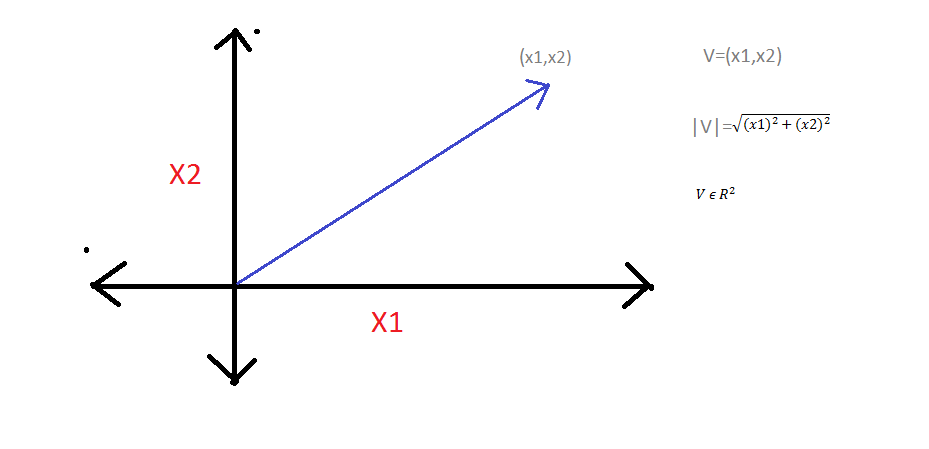# Essential Mathematical Concepts for Machine Learning-Linear AlgebraWe will start with Linear Algebra basics. I might be posting 2 or 3 more articles on Linear Algebra to cover those topics which are relevant to machine learning. The topics to be discussed are listed as follows:-

In this particular article, we will start with Linear Algebra basics. I might be posting 2 or 3 more articles on Linear Algebra to cover those topics which are relevant to machine learning. The topics to be discussed are listed as follows:-

1. Vectors
2. Vector Spaces and Subspaces
3. Linear Span
4. Column Space
5. Null Space

*Vectors:- *It is an object that has both magnitudes as well as the direction. Geometrically, we can visualize a vector as a directed line segment whose length gives the magnitude of the vector and arrow gives the direction.In machine learning, we use vectors to represent features and labels of the input data like age, salary, etc. Here in this example, V is a two- dimensional vector from the field of Real Numbers.

*Vector Space:- *A vector space is a set V of vectors on which the vector addition and vector multiplication are defined such that,

1. (V,+) is an abelian group.
2. The dot product between real numbers and vector v is defined in such a way that for all “a” belongs to Real numbers and v belongs to the vector space V such that a.v should belong to the vector space V.
3. For all ‘a’ belongs to real numbers (R) and v,w belongs to V(vector space), a(v+w)=a.v+a.w
4. For all a,b belongs to R and v belongs to V, (a+b).v = a.v+b.v
5. For all a,b belongs to R and v belongs to V, (ab).v=a.(b.v)
6. (Unitary law) 1 belongs to R and v belongs to V, 1.v belongs to V.

## Linear Algebra: The hidden engine of machine learning

The hidden engine of machine learning. Algebra is firstly taken from a book, written by Khwarizmi(780-850 CE), which is about calculation and equations.

## Linear Algebra Concepts (For Machine Learning)

Basic Mathematical Concepts for Machine Learning. Machine learning at its base is complete and pure mathematics and logic.

## What is Supervised Machine Learning

What is neuron analysis of a machine? Learn machine learning by designing Robotics algorithm. Click here for best machine learning course models with AI

## Pros and Cons of Machine Learning Language

AI, Machine learning, as its title defines, is involved as a process to make the machine operate a task automatically to know more join CETPA

## Appendix: Linear Algebra

These notes are intended to provide a “fast” collection of theoretical definitions, theorems, and concepts for Linear Algebra as a refresher only